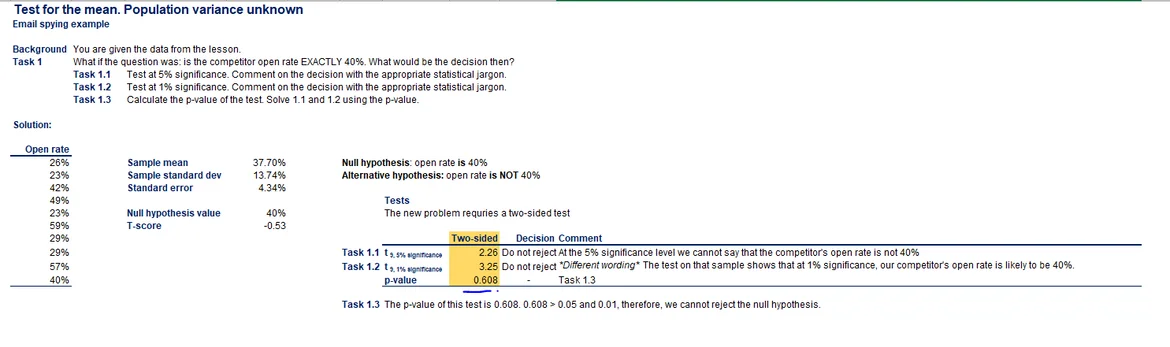01 Dec 2022

Posted on:

30 Sept 2022

3

# How to get the p-value of 0.608

Please show me how to get the p-value of 0.608 in the excel file "5.6.Test-for-the-mean.Population-variance-unknown-exercise-solution". I try to calculate in https://www.socscistatistics.com/ but it shows different results. ThanksPosted on:

06 Nov 2022

0

Exactly. I get two different p-values 0.50177 and 0.010159 for 5% and 1% Significance levels.

Super learner
Posted on:

01 Dec 2022

0

In the p-value video we see that for 2-sided tests, the p-value for a one-sided test gets multiplied by 2. In the example, the p-value for a one-sided test is .304, so multiplied by 2 we get .608. I also got the same result in the calculator using a T score of 0.53, 9 degrees of freedom, a significance level of .05 (although this seems irrelevant), and a two-tailed hypothesis option.